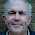An oldie but a goodie

Recently we've been having a look at multiplication in Year 4 so we got onto the "Area and Perimetre Robot" activity. It's an old faithful but didn't the kids love it!

The idea is to use rectangular shapes to make up a robot. Then the kids have to do a series of calculations to work out the area of each shape before they can calculate the total area of the robot.

Step two is to work out the perimeter.

One of the first questions from a keen student was, "Can I use triangles in my robot?"

"Well," I replied, "Do you know how to work out the area of a triangle?"

"Sure - base times height divided by 2!"

"Okay, then you can use triangles."

It sure is going to be an interesting adventure when we get to work out the perimeter of that robot. Look out Pythagoras - here we come!

Here's a few examples from the Year 4 kids in Capitano Amazing's class - they look great! Sadly, the calculations were not really dark enough to be picked up in the photo.

1.I can see why the "Area and Perimeter Robot" activity was popular. Adding some art to maths would be more attractive to Year 4. I was able to make out their calculations on the pictures.

The person who asked if it was possible to use triangles was interesting, particularly as he knew how to calculate the area of the triangle. I wonder if he discovered the disadvantage when trying to accurately calculate the perimeter of the robot? The sine equation would be a little advanced. :)

@RossMannell

2.Yep - we had a bit of a talk about Pythagorian triangles and decided to use an "approximation" for the length rather than √2. A bit of a compromise but appropriate for Year 4 in practical terms.

Any comments you would like to make?